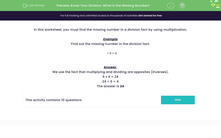# Use Multiplication as the Inverse Operation of Division

In this worksheet, students will find the missing number in a division fact by using multiplication.Key stage:  KS 2

Curriculum topic:   Number: Multiplication and Division

Curriculum subtopic:   Use Multiplication/Division Facts

Popular topics:   Multiplication worksheets, Division worksheets

Difficulty level:#### Worksheet Overview

In this activity, we will be finding the missing number in a division fact by using multiplication.

Example

Find the missing number in the division fact.

÷ 6 = 4

We use the fact that multiplying and dividing are opposites (inverses).

6 x 4 = 24

24 ÷ 6 = 4

We can always check it to see if it works.

24 ÷ 6 = 4

It's correct!Let's try some questions now.

### What is EdPlace?

We're your National Curriculum aligned online education content provider helping each child succeed in English, maths and science from year 1 to GCSE. With an EdPlace account you’ll be able to track and measure progress, helping each child achieve their best. We build confidence and attainment by personalising each child’s learning at a level that suits them.

Get started#### Popular Maths topics

••••